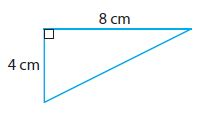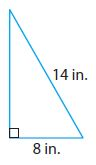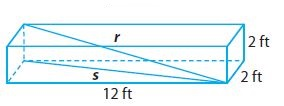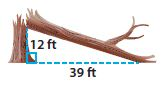Homework Explained - Math Practice 101Dear guest, you are not a registered member. As a guest, you only have read-only access to our books, tests and other practice materials.

As a registered member you can:

Registration is free and doesn't require any type of payment information. Click here to Register.
Go to page:
Chapter 12:The Pythagorean Theorem; Lesson 1: The Pythagorean Theorem

### 12.1 Independent Practice

Find the length of the missing side of each triangle. Round your answers to the nearest tenth.

• Question 4•  cm
• Question 5•  in.
• Question 6

The diagonal of a rectangular big-screen TV screen measures 152 cm. The length measures 132 cm. What is the height of the screen?

•  cm
• Question 7

Dylan has a square piece of metal that measures 10 inches on each side. He cuts the metal along the diagonal, forming two right triangles. What is the length of the hypotenuse of each right triangle to the nearest tenth of an inch?

•  in.
• Question 8

Represent Real-World Problems A painter has a 24-foot ladder that he is using to paint a house. For safety reasons, the ladder must be placed at least 8 feet from the base of the side of the house. To the nearest tenth of a foot, how high can the ladder safely reach?

•  ft
• Question 9

What is the longest flagpole (in whole feet) that could be shipped in a box that measures 2 ft by 2 ft by 12 ft?•  ft
• Question 10

Sports American football fields measure 100 yards long between the end zones, and are $$53 \frac{1}{3}$$yards wide. Is the length of the diagonal across this field more or less than 120 yards? Explain.

• Question 11

Justify Reasoning A tree struck by lightning broke at a point 12 ft above the ground as shown. What was the height of the tree to the nearest tenth of a foot? Explain your reasoning.•  ft

Yes, email page to my online tutor. (if you didn't add a tutor yet, you can add one here)